Publicité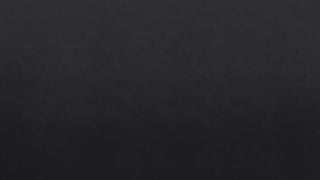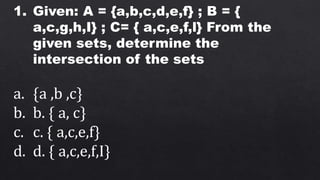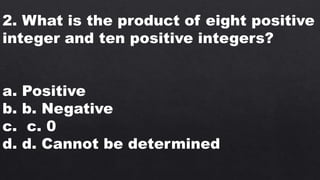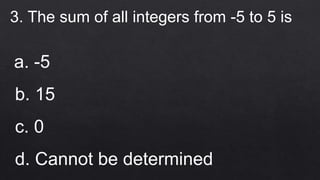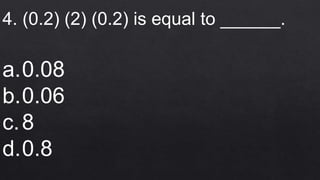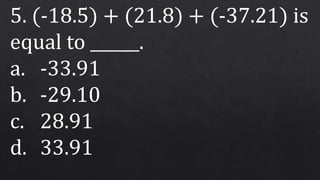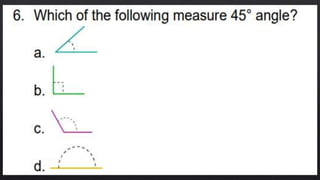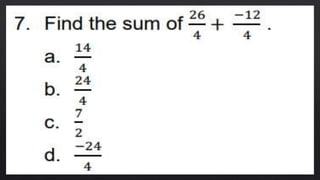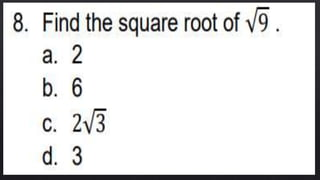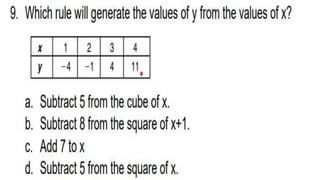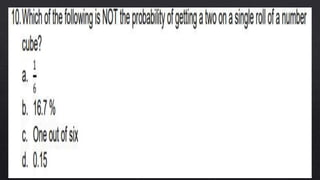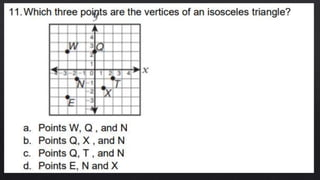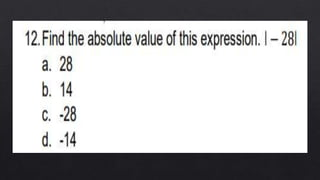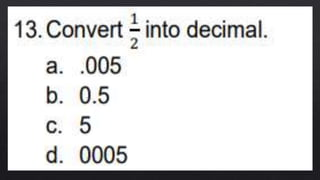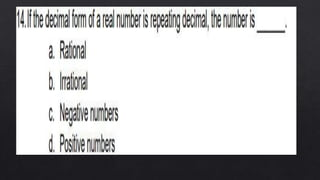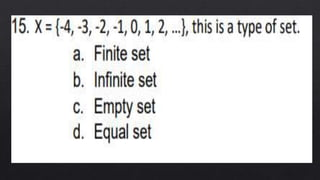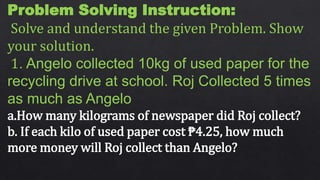1 sur 24
Publicité

### Diagnostic.pptx

1. 1. Given: A = {a,b,c,d,e,f} ; B = { a,c,g,h,I} ; C= { a,c,e,f,l} From the given sets, determine the intersection of the sets a. {a ,b ,c} b. b. { a, c} c. c. { a,c,e,f} d. d. { a,c,e,f,I}
2. 2. What is the product of eight positive integer and ten positive integers? a. Positive b. b. Negative c. c. 0 d. d. Cannot be determined
3. 3. The sum of all integers from -5 to 5 is a. -5 b. 15 c. 0 d. Cannot be determined
4. 4. (0.2) (2) (0.2) is equal to ______. a.0.08 b.0.06 c.8 d.0.8
5. 5. (-18.5) + (21.8) + (-37.21) is equal to ______. a. -33.91 b. -29.10 c. 28.91 d. 33.91
6. Problem Solving Instruction: Solve and understand the given Problem. Show your solution. 1. Angelo collected 10kg of used paper for the recycling drive at school. Roj Collected 5 times as much as Angelo a.How many kilograms of newspaper did Roj collect? b. If each kilo of used paper cost ₱4.25, how much more money will Roj collect than Angelo?
Publicité